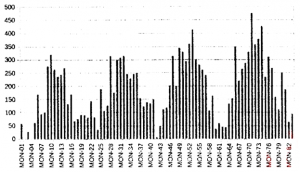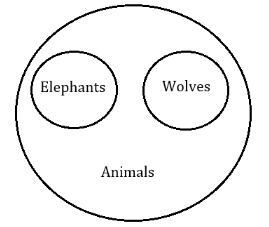## HCU PHD CS MAY 2019

 Question 1
Consider the polynomial p(x)=c0 + c1x + c2x^2 + c3x^3, where no coefficient is 0. The minimum number of multiplications required to evaluate p on an input x is
 A 6 B 4 C 3 D 8
Engineering-Mathematics       Combinatorics
Question 1 Explanation:
Given, p(x)=c0 + c1x + c2x^2 + c3 x^3
For minimum number of multiplications, lets modify above equation,
p(x)= c0 + x(c1 + c2x + c3 x^2)
=c0+x(c1+x(c2+c3x)
=c0+x*(c1+x*(c2+c3*x)
Hence minimum number of multiplications required is 3
 Question 2
If S={x|0 < x < 12}, M={x|1 < x < 9} and N={x|0 < x< 5}, find M' ∩ N'
 A {x|9 ≤ x < 5} B {x|9 < x < 12} C {x|0 < x < 12} D {x|5 < x < 9}
Engineering-Mathematics       Set-Theory
Question 2 Explanation:
S = Total range = {x| 0 Now,
M = {x| 1 M’ = {x| 0 and
N = {x| 0 N’ = {x| 5≤x<12}
Now, clearly the intersection range of M’ and N’ is,
{x| 9≤x<12}
 Question 3
Let E, F and G be finite sets and let A = (E∩ F) - (F ∩ G) and B = (E - (E ∩ G)) - (E - F). Which one of the following is true?
 A A ⊂ B B S ⊂ A C A = B D None of the above
Engineering-Mathematics       Set-Theory
Question 3 Explanation:Now,
A = (E∩F) - (F∩G)
= (2,5) - (5,6)
= {2}
Also,
B = (E - (E∩G)) - (E - F)
= ({1,2,4,5} - {4,5}) - (1,4)
= (1,2) - (1,4)
= {2}
Hence A = B
 Question 4
If the word FORGET is encoded as DPPHCU, then THINK is encoded as
 A VGKMM B RIILI C RIGOI D RIGOR
Aptitude       Numerical
Question 4 Explanation:Question 5
Seven (distinct) road accidents occurred in a week. What is the probability that they all occurred on the same day?
 A 7^-6 B 7^-2 C 2^-7 D 7^-7
Engineering-Mathematics       Probability
Question 5 Explanation:
For every car accident we can choose a day in 7 ways. So total no. of ways in which 7 car accident can be assigned to 7 days = 7 × 7 × 7 × 7 × 7 × 7 × 7 = 77
Probability of accident happening on one day = 1/77
But there are 7 days in which we have to choose 1 day, in which all accidents are happening. Hence required probability is
7C1/77 = 7/77 = 1/76
 Question 6
What is the maximum number of different Boolean functions involving n Boolean variables?
 A n^2^n B 2^n C 2^2^n D n^2
Digital-Logic-Design       Boolean-Function
Question 6 Explanation:
No. of rows possible with n boolean variables is 2^n, and each row can have two functions possible . Hence the total number of boolean functions possible is 2^(2^n).
 Question 7

Given below is a chart of rainfall amounts in Quinquinox city on a distant planet Quinox revolving around an equally distant Star. It has its own months and years. Year is, of course, the time takenin their own units of measurement to revolve once around their Star.

Examine the chart carefully and answer QuestionHow many months are there in a year on Quinox?
 A 10 B 20 C 28 D Not possible from the data given
 Question 8

Given below is a chart of rainfall amounts in Quinquinox city on a distant planet Quinox revolving around an equally distant Star. It has its own months and years. Year is, of course, the time taken in their own units of measurement to revolve once around their Star.

Examine the chart carefully and answer QuestionIf climate is classified into the following three categories,

QNd Seasonal rainfall with wet season being short

QNe Uniform rainfall throughout the year

QNm Seasonal rainfall with wet season being long

Which category describes the climate of Quinquinox?
 A QNm B QNe C QNd D Not possible from the data given
 Question 9If the year on Quinox starts with Mon-Ol (which is also the first data point), which month in a year is the wettest?
 A 18 B 12 C 8 D 5
 Question 10
Let S be a set of n elements. The number of ordered pairs in the largest and the smallest equivalence relations on S are
 A n^2 and n B n^2 and 0 C n(n+1)/2 and n D n(n+1)/2 and 0
Engineering-Mathematics       Set-Theory
Question 10 Explanation:
For the relation to be equivalence ,it should be
->Reflexive
->Symmetric
->Transitive
The smallest equivalence relation is on size n, which contains all diagonal elements.
The largest equivalence relation is the maximum size of relation itself ,i.e. n^2.
 Question 11

Here is a puzzle: find a number x such that x leaves a remainder of 9 when divided by 10, a remainder of 8 when divided by 9, a remainder of 7 when divided by 8, ... , a remainder of 2 when divided by 3 and a remainder of 1 when divided by 2.

How many such numbers are there?
 A 0 B Exactly 1 C 2``` D Infinite
Aptitude       Numerical
Question 11 Explanation:
The lowest number divisible by all the numbers 1 to 10. So, for minimum value of N,
===>N+1=2520 (LCM of 1,2,3,....10)
or,N=2519
This property is satisfied by the number N=2520∗K−1, where K is a positive integer.
 Question 12
Car A goes 200 Kms at an average speed of 40 Kmph in one direction and returns to the starting point covering the distance of 200 Kms at an average speed of 50 Kmph. Another Car B goes 200 Kms at an average speed of 45 Kmph and does the return journey of 200 Kms also at an average speed of 45 Kmph. Which statement is TRUE about the two cars A and B?
 A Car A takes less time than Car B B Car B takes less time than Car A C Both Cars A and B take the same time D Cannot be determined from the data given
Aptitude       Numerical
Question 12 Explanation:
Time taken by car A for complete journey = t1 + t2
= 200/40 + 200/50
= 5 + 4
= 9 hr
Time taken by car B for complete journey = t 1 + t2
= 200/45 + 200/45
= 8.88 hr
Car B takes less time than car A.
 Question 13Which of the following is correctly represented by the Venn diagram above?
 A Elephants, Wolves, Animals B Dogs, Cats, Pets C Pigeons, Dogs, Birds D Tables, Chairs, Furniture
Aptitude       Numerical
Question 13 Explanation:
Let’s check option wise,
A) Can’t be the answer because all Elephants and Wolves are animals hence the diagram would be,B) Yes, True. Because there are some dogs which are pets and there are some cats which are pets. Not all of them are pets. So in the given diagram naming will be,C)Can’t be the answer because Dogs are not selected to be pigeons and birds.
D)Can’t be the answer because all Tables and chairs are furniture. Hence diagram will be same like (A).
 Question 14

Questions 14 - 16 are based on the Venn diagram below. S represents all integers between 1 and 30, P represents prime numbers between 1 and 30, M represents multiples of 3 between 1 and 30, and F represents all factors of 30.If G = P ∩ M ∩ F, then
 A G = {3} B G = {3.5} C G = {1} D G={1,3,5}
Engineering-Mathematics       Set-Theory
Question 14 Explanation:
S = 1, 2, 3, 4, …, 30
P = 2,3,5,7,11,13,17,19,23,29
M = 3,6,9,12,15,18,21,24,27,30
F = 1,2,3,5,6,10,15,30
P∩M∩F = {3}
 Question 15

Questions 14 - 16 are based on the Venn diagram below. S represents all integers between 1 and 30, P represents prime numbers between 1 and 30, M represents multiples of 3 between 1 and 30, and F represents all factors of 30.What are the numbers in F but not in P or M?
 A 1, 10 B Only 1 C Only 10 D 1,3,10
Engineering-Mathematics       Set-Theory
Question 15 Explanation:
S = 1, 2, 3, 4, …, 30
P = 2,3,5,7,11,13,17,19,23,29
M = 3,6,9,12,15,18,21,24,27,30
F = 1,2,3,5,6,10,15,30
F - (P∪M) = {1,2,3,5,6,10,15,30} - {2,3,5,6,7,9,11,12,13,15,17,18,19,21,23,24,27,29,30} = {1,10}
 Question 16

Questions 14 - 16 are based on the Venn diagram below. S represents all integers between 1 and 30, P represents prime numbers between 1 and 30, M represents multiples of 3 between 1 and 30, and F represents all factors of 30.What is the cardinality of P ∩ F?
 A 1 B 2 C 3 D 4
Engineering-Mathematics       Set-Theory
Question 16 Explanation:
S = 1, 2, 3, 4, …, 30
P = 2,3,5,7,11,13,17,19,23,29
M = 3,6,9,12,15,18,21,24,27,30
F = 1,2,3,5,6,10,15,30
P∩F = {2,3,5}
Hence cardinality of P∩F = 3
 Question 17

When looking back on any kind of movement or revolution, one always likes to point to a beginning: "It began right there - it all started with so-and-so's famous speech ... " If structured

programming can be thought of as a revolution, then surely Dijkstra's land-mark paper, "Programming Considered as a Human Activity," published in 1965, marks its beginning. Virtually

the entire gospel of structured programming is contained in a few short pages: the arguments

against goto statements, the notion of top-down design, the emphasis on program correctness

and quality (or "elegance," as Dijkstra prefers to call it), and the strong argument against programs that modify themselves.

In addition to these fundamental concepts, there are some rather classic phrases that first appeared in this paper, and that have popped-up in dozens of subsequent articles and books. For

example, when discussing the "divide and conquer" approach characterizing top-down design,

Dijkstra admits, "I have only a very small head, ,and must live with it." What seems obvious to us today was a rather novel idea in 1965: the idea that while computers were - and still are

- getting faster and more powerful, human beings weren't.

This theme is repeated again and again, and is essentially the entire subject matter of Dijkstra's

1972 speech, "The Humble Programmer." ... Once you do read it, you can see why Dijkstra has

the reputation he does. His writing is succinct and yet convincing. Reading Dijkstra, someone

said, has been compared to eating marzipan - it's best to take very small bites, chew slowly,

and digest the mouthful before moving on to the next bite.

What is said to have begun with Dijkstra's landmark paper, "Programming Considered as a Human Activity" in 1965?
 A Structured programming B Programs which modify themselves that eventually led to viruses C High salaries to programmers D Computer revolution
Aptitude       Verbal
Question 17 Explanation:
From the line
“ If structured programming can be thought of as a revolution, then surely Dijkstra's land-mark paper, "Programming Considered as a Human Activity," published in 1965, marks its beginning. ” , clearly the answer is (A) .
There are 17 questions to complete.

Register Now